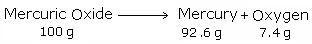# Lavoisier's Law of Conservation of Mass

$$\newcommand{\vecs}{\overset { \rightharpoonup} {\mathbf{#1}} }$$ $$\newcommand{\vecd}{\overset{-\!-\!\rightharpoonup}{\vphantom{a}\smash {#1}}}$$$$\newcommand{\id}{\mathrm{id}}$$ $$\newcommand{\Span}{\mathrm{span}}$$ $$\newcommand{\kernel}{\mathrm{null}\,}$$ $$\newcommand{\range}{\mathrm{range}\,}$$ $$\newcommand{\RealPart}{\mathrm{Re}}$$ $$\newcommand{\ImaginaryPart}{\mathrm{Im}}$$ $$\newcommand{\Argument}{\mathrm{Arg}}$$ $$\newcommand{\norm}{\| #1 \|}$$ $$\newcommand{\inner}{\langle #1, #2 \rangle}$$ $$\newcommand{\Span}{\mathrm{span}}$$ $$\newcommand{\id}{\mathrm{id}}$$ $$\newcommand{\Span}{\mathrm{span}}$$ $$\newcommand{\kernel}{\mathrm{null}\,}$$ $$\newcommand{\range}{\mathrm{range}\,}$$ $$\newcommand{\RealPart}{\mathrm{Re}}$$ $$\newcommand{\ImaginaryPart}{\mathrm{Im}}$$ $$\newcommand{\Argument}{\mathrm{Arg}}$$ $$\newcommand{\norm}{\| #1 \|}$$ $$\newcommand{\inner}{\langle #1, #2 \rangle}$$ $$\newcommand{\Span}{\mathrm{span}}$$$$\newcommand{\AA}{\unicode[.8,0]{x212B}}$$

With the development of more precise ideas on elements, compounds and mixtures, scientists began to investigate how and why substances react. French chemist A. Lavoisier laid the foundation to the scientific investigation of matter by describing that substances react by following certain laws. These laws are called the laws of chemical combination. These eventually formed the basis of Dalton's Atomic Theory of Matter.

## Law of Conservation of Mass

According to this law, during any physical or chemical change, the total mass of the products remains equal to the total mass of the reactants.The law of conservation of mass is also known as the "law of indestructibility of matter."

##### Example $$\PageIndex{1}$$

If heating 10 grams of $$\ce{CaCO3}$$ produces 4.4 g of $$\ce{CO2}$$ and 5.6 g of $$\ce{CaO}$$, show that these observations are in agreement with the law of conservation of mass.

###### Solution
• Mass of the reactants: $$10 \,g$$
• Mass of the products: $$4.4 \,g+ 5.6\, g = 10\, g$$.

Because the mass of the reactants is equal to the mass of the products, the observations are in agreement with the law of conservation of mass.

Lavoisier's Law of Conservation of Mass is shared under a CC BY-NC-SA 4.0 license and was authored, remixed, and/or curated by Binod Shrestha.